## Math Worksheets

#####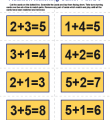Matching Game 1 thru 5

Players will take turns matching cards which have addition problems
for the numbers 1 through 5

#####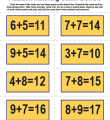Match Game 2 thru 9

Students will take turns matching card sets which have addition problems for the numbers 2 through 9.

#####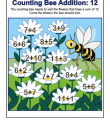Counting Bee Addition: 12

Your busy little bee will learn some early addition skills with this colorful worksheet. Student must identify problems which sum’s are 12.

#####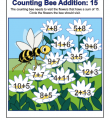Counting Bee Addition: 15

This honey of a worksheet will help your little one learn early addition skills. Student must identify problems which sum’s equal 15.

#####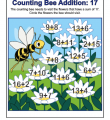Counting Bee Addition: 17

Add some addition practice to your busy bee’s schedule with this colorful math worksheet. Student must identify problems which sum’s equal 17.

### SUBTRACTION

#####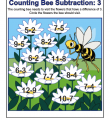Counting Bee Subtraction: 3

Add some subtraction practice to your busy little bee’s schedule with this colorful math worksheet. Student must identify all problems which a difference of 3.

#####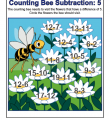Counting Bee Subtraction: 5

Your busy little bee will learn early subtraction skills with this colorful worksheet. Student must identify problems with a difference of 5.

#####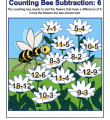Counting Bee Subtraction: 6

This honey of a worksheet will help your little one learn early subtraction skills. Students identify problems with a difference of 6.

#####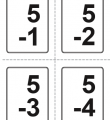Subtraction Flash Cards

Help your student improve her subtraction skills with this set of subtraction flash cards.

### MULTIPLICATION

#####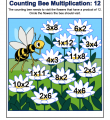Counting Bee Multiplication: 12

Help the bee find the product 12. Where could it bee? Students will choose all flowers with the correct answers.

#####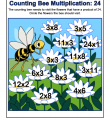Counting Bee Multiplication: 24

The bee is on the hunt for problems with the product 24. Students will find all flowers with the correct answers.

#####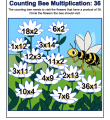Counting Bee Multiplication: 36

Help the bee find the product 36. There’s a whole swarm of possibilities in this colorful worksheet!

### DIVISION

#####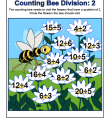Counting Bee Division: 2

In this division worksheet students search for quotients of 2.

#####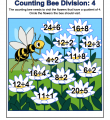Counting Bee Division: 4

Help the bee find the quotient 4.

#####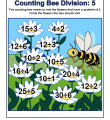Counting Bee Division: 5

This worksheet poses a honey of a problem…
where is the quotient 5?

### FACT FAMILIES

#####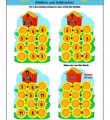Sunflowers and Birdies Fact Families: Addition and Subtraction

This very colorful worksheet will give some great practice for adding and subtracting numbers in fact families.

#####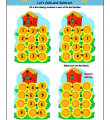Sunflowers and Birdies Fact Families: Let’s Add and Subtract

Practice adding and subtracting numbers in fact families with this colorful worksheet.

#####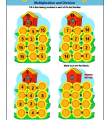Sunflowers and Birdies Fact Families: Multiplication and Division

This very colorful worksheet will give some great practice for multiplying and dividing numbers in fact families.

#####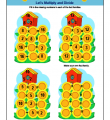Sunflowers and Birdies Fact Families: Let’s Multiply and Divide

Give your students some great practice multiplying and dividing numbers in fact families with this very colorful worksheet.